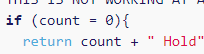# What is happening here? (Counting Cards)

I was trying to solve the counting cards challenge on FCC the link is Counting Cards FCC
I successfully typed a switch statement which is able to change the value of count variable according to given sequence correctly only if after the switch statement i type `return count;`
But to add strings Hold and Bet in front of count variable value i used if and if else statements which returns undefined every-time. So the problem is if i use `return count;` after the switch statement then i will not be able to use if statements because the function will end there and if i use if and if else statements after the switch statement then the function return undefined every time why what’s the problem in this code? Here is the code -

``````let count = 0;

function cc(card) {
// Only change code below this line
switch(card){
case 2:
count+=1;
break;
case 3:
count+=1;
break;
case 4:
count+=1;
break;
case 5:
count+=1;
break;
case 6:
count+=1;
break;
case 10:
count -= 1;
break;
case 'J':
count -= 1;
break;
case 'Q':
count -= 1;
break;
case 'K':
count -= 1;
break;
case 'A':
count -= 1;
break;
}

return count; // This is working fine
//cc(2); cc(3); cc(7); cc('K'); cc('A'); IS RETURNING 0
//cc(10); cc("J"); cc("Q"); cc('K'); cc('A'); IS RETURNING -5 etc...

/*
THIS IS NOT WORKING AT ALL ONLY RETURNING UNDEFINED EVERYTIME WHY?
if (count = 0){
return count + " Hold";
}
else if (count > 0){
return count + " Bet";
}
else if (count < 0){
return count + " Hold";
}
*/
// Only change code above this line
}
``````

The problem could be here:It is if( count === 0). You need to put == or === in place of just =.
Just one = means assignment, not condition check!

2 Likes

Thank you !
This solved the problem.